Calculus 3 : Dot Product

Example Questions

Example Question #416 : Vectors And Vector Operations

Solve: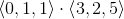Explanation:

The dot product of two vectors is given by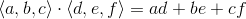Using this, we get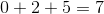Example Question #417 : Vectors And Vector Operations

Solve: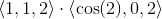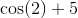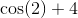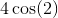Explanation:

The dot product of two vectors is given byUsing this, we get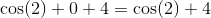Example Question #418 : Vectors And Vector Operations

Solve: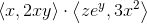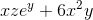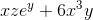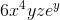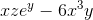Explanation:

The dot product of two vectors is given by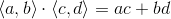Example Question #419 : Vectors And Vector Operations

Find the dot product of the vectors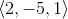and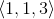Explanation:

The formula for the dot product is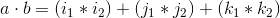. Using the given vectors, we get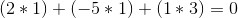.

Example Question #420 : Vectors And Vector Operations

Compute the dot product between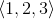and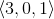.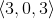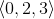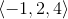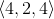Explanation:

The formula for the dot product is. Using the two given vectors and solving,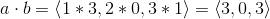.

Example Question #101 : Dot Product

Solve: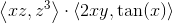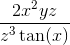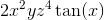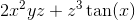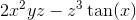Explanation:

The dot product of two vectors is given byExample Question #102 : Dot Product

Solve: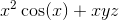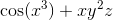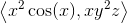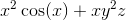Explanation:

The dot product of two vectors is found by adding the product of the corresponding components. (For example,.)

For our vectors, we getExample Question #103 : Dot Product

Solve: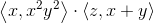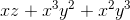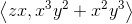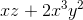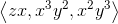Explanation:

The dot product of two vectors is given by the sum of the products of the corresponding components (for example,).

So, we getExample Question #104 : Dot Product

Solve: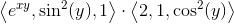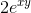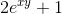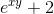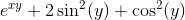Explanation:

The dot product of two vectors is given bySo, we getWe used a Pythagorean trigonometric identity to simplify.

Example Question #105 : Dot Product

Solve: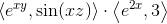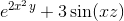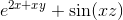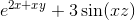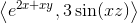The dot product of two vectors is given by the sum of the products of the corresponding components (ex.)